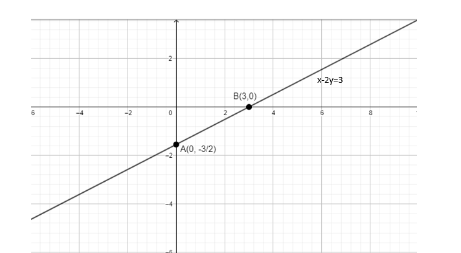QuestionAnswers

# Draw the graph of the line $x-2y=3$ . From the graph, find the coordinates of the point when(i) $x=-5$ (ii) $y=0$ (a) (-5, - 4) and (3, 0)(b) (-4, -5) and (3, 0)(c) (-4, 3) and (5, 0)(d) (-5, 0) and (-4, 0)

Hint: In order to draw the graph for any line equation, the best possible way is to find the two coordinates and join those two points. This way we can easily get the graph. In order to get the coordinates, we need to take the following two steps: (i) replace $y=0$ in the given equation and find the coordinates of a point (ii) replace $x=0$ in the given equation and find the coordinates of the other point. Here, we will follow the same trick, firstly we will find the coordinates of the two points using the tricks mentioned in (i) and (ii) and connect the points which will give us the required graph.

Here, we have to find the graph of the given line equation $x-2y=3......................(i)$ . Now, we will replace the value of x and y with ‘zero’, one by one, and find the required two coordinates, i. e., point A and point B (let us say).
Replacing the value of x with ‘zero’ in equation (i), we get,
$\Rightarrow 0-2y=3$
$\Rightarrow -2y=3$
$\Rightarrow 2y=-3$
$\therefore y=\dfrac{-3}{2}$
Hence when $x=0$ in the above equation (i), the value of y is $-\dfrac{3}{2}$ . So, the point is $\text{A}\left( 0,\dfrac{-3}{2} \right)$ .
Similarly, now, we place the value of y with ‘zero’ in the above equation (i), we get,
$\Rightarrow x-2\times 0=3$
$\Rightarrow x-0=3$
$\therefore x=3$
Hence when $y=0$ in the above equation (i), the value of x is 3. So, the point is $\text{B}\left( 3,0 \right)$ .
Now, we need to plot these points $\text{A}\left( 0,\dfrac{-3}{2} \right)$ and $\text{B}\left( 3,0 \right)$ in the x – y plane and join these points, in order to obtain the required graph for the equation $x-2y=3$ .Now, to find the coordinates of the point under a given condition, we need to simply replace that value in the given equation (i), so
(i) Replace the value of x with -5 in the given equation (i), we get,
$\Rightarrow -5-2y=3$
$\Rightarrow -2y=3+5$
$\Rightarrow -2y=8$
$\Rightarrow 2y=-8$
$\Rightarrow y=\dfrac{-8}{2}$
$\therefore y=-4$
Hence when $x=-5$ in the above equation (i), the value of y is -4. So, the required coordinates of point are $\left( -5,-4 \right)$ .
(ii) Replace the value of y with 0 in the given equation (i), we get,
$\Rightarrow x-2\times 0=3$
$\Rightarrow x-0=3$
$\therefore x=3$
Hence when $y=0$ in the above equation (i), the value of x is 3. So, the required coordinates of point are $\left( 3,0 \right)$ .
So, the correct answer is “Option A”.

Note: Students normally make a mistake while replacing the values in the equation and solving the equation and also while plotting the point in the graph. One other alternate method, to find the coordinates at some given condition is to simply solve the given condition and the given equation as shown below:
(i) when $x=-5$ , we simply need to solve this equation and equation (i),
$x-2y=3............(ii)$
$x=-5........(iii)$
Now, subtracting equation (iii) from (ii), we get,
\begin{align} & x-2y=3 \\ & -x-0y=5 \\ & \underline{\text{ }} \\ & -2y=8 \\ \end{align}
$\Rightarrow 2y=-8$
$\Rightarrow y=\dfrac{-8}{2}$
$\Rightarrow y=-4$
Hence when $x=-5$ in the above equation (i), the value of y is -4. So, the required coordinates of point are $\left( -5,-4 \right)$ .

(i) when $y=0$ , we simply need to solve this equation and equation (i),
$x-2y=3............(ii)$
$y=0........(iii)$
Now, multiplying equation (iii) by 2, we get,
$2y=0..............(iv)$
Adding equation (ii) from (iv), we get,

\begin{align} & x-2y=3 \\ & 0x+2y=0 \\ & \underline{\text{ }} \\ & x=3 \\ \end{align}
Hence when $y=0$ in the above equation (i), the value of x is 3. So, the required coordinates of point are $\left( 3,0 \right)$ .